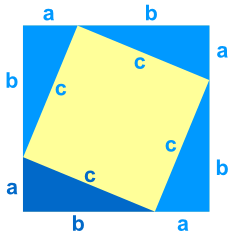# Pythagorean Theorem Algebra Proof

## What is the Pythagorean Theorem?

You can learn all about the Pythagorean Theorem, but here is a quick summary:The Pythagorean Theorem says that, in a right triangle, the square of a (which is a×a, and is written a2) plus the square of b (b2) is equal to the square of c (c2):

a2 + b2 = c2

## Proof of the Pythagorean Theorem using Algebra

We can show that a2 + b2 = c2 using Algebra

Take a look at this diagram ... it has that "abc" triangle in it (four of them actually):## Area of Whole Square

It is a big square, with each side having a length of a+b, so the total area is:

A = (a+b)(a+b)

## Area of The Pieces

Now let's add up the areas of all the smaller pieces:

First, the smaller (tilted) square has an area of: c2

Each of the four triangles has an area of:ab2
So all four of them together is:4ab2 = 2ab

Adding up the tilted square and the 4 triangles gives:A = c2 + 2ab

## Both Areas Must Be Equal

The area of the large square is equal to the area of the tilted square and the 4 triangles. This can be written as:

(a+b)(a+b) = c2 + 2ab

NOW, let us rearrange this to see if we can get the pythagoras theorem: